Courses

# Linear Algebra and Matrices - Mathematical Methods of Physics, UGC - NET Physics Physics Notes | EduRev

## Physics : Linear Algebra and Matrices - Mathematical Methods of Physics, UGC - NET Physics Physics Notes | EduRev

The document Linear Algebra and Matrices - Mathematical Methods of Physics, UGC - NET Physics Physics Notes | EduRev is a part of the Physics Course Physics for IIT JAM, UGC - NET, CSIR NET.
All you need of Physics at this link: Physics

Introduction

The mathematical idea of a vector plays an important role in many areas of physics.

• Thinking about a particle traveling through space, we imagine that its speed and direction of travel can be represented by a vector v in 3-dimensional Euclidean space R3. Its path in time t might be given by a continuously varying line — perhaps with self-intersections — at each point of which we have the velocity vector v(t).
• A static structure such as a bridge has loads which must be calculated at various points. These are also vectors, giving the direction and magnitude of the force at those isolated points
• In the theory of electromagnetism, Maxwell’s equations deal with vector ﬁelds in 3-dimensional space which can change with time. Thus at each point of space and time, two vectors are speciﬁed, giving the electrical and the magnetic ﬁelds at that point.
• Given two diﬀerent frames of reference in the theory of relativity, the transformation of the distances and times from one to the other is given by a linear mapping of vector spaces.
• In quantum mechanics, a given experiment is characterized by an abstract space of complex functions. Each function is thought of as being itself a kind of vector. So we have a vector space of functions, and the methods of linear algebra are used to analyze the experiment.

Looking at these ﬁve examples where linear algebra comes up in physics, we see that for the ﬁrst three, involving “classical physics”, we have vectors placed at diﬀerent points in space and time. On the other hand, the ﬁfth example is a vector space where the vectors are not to be thought of as being simple arrows in the normal, classical space of everyday life. In any case, it is clear that the theory of linear algebra is very basic to any study of physics.

But rather than thinking in terms of vectors as representing physical processes, it is best to begin these lectures by looking at things in a more mathematical, abstract way. Once we have gotten a feeling for the techniques involved, then we can apply them to the simple picture of vectors as being arrows located at diﬀerent points of the classical 3-dimensional space.

Basic Deﬁnitions Deﬁnition.

Let X and Y be sets. The Cartesian product X × Y , of X with Y is the set of al l possible pairs (x, y ) such that x ∈ X and y ∈ Y .

Deﬁnition. : A group is a non-empty set G, together with an operation1, which is a mapping ‘ · ’ : G × G → G, such that the fol lowing conditions are satisﬁed.

1. For al l a, b, c ∈ G, we have (a · b) · c = a · (b · c),

2. There exists a particular element (the “neutral” element), often cal led e in group theory, such that e · g = g · e = g, for al l g ∈ G.

3. For each g ∈ G, there exists an inverse element g−1 ∈ G such that g · g−1 = g−1 · g = e.

If, in addition, we have a · b = b · a for al l a, b ∈ G, then G is cal led an “Abelian” group.

Deﬁnition. A ﬁeld is a non-empty set F , having two arithmetical operations, denoted by ‘+’ and ‘·’, that is, addition and multiplication2. Under addition, F is an Abelian group with a neutral element denoted by ‘0’. Furthermore, there is another element, denoted by ‘1’, with 1 = 0, such that F \ {0} (that is, the set F , with the single element 0 removed) is an Abelian group, with neutral element 1, under multiplication. In addition, the distributive property holds:

a · (b + c) = a · b + a · c and (a + b) · c = a · c + b · c, for al l a, b, c ∈ F .

The simplest example of a ﬁeld is the set consisting of just two elements {0, 1} with the obvious multiplication. This is the ﬁeld Z/2Z. Also, as we have seen in the analysis lectures, for any prime number p ∈ N, the set Z/pZ of residues modulo p is a ﬁeld.

The following theorem, which should be familiar from the analysis lectures, gives some elementary general properties of ﬁelds.

Theorem 1. Let F be a ﬁeld. Then for all a, b ∈ F , we have:

1. a · 0 = 0 · a = 0,

2. a · (−b) = −(a · b) = (−a) · b,

3. −(−a) = a,

4. (a−1)−1 = a, if a = 0,

5. (−1) · a = −a,

6. (−a) · (−b) = a · b,

7. a · b = 0 ⇒ a = 0 or b = 0.

 1 - The operation is usually called “multiplication” in abstract group theory, but the sets we will deal with are also groups under “addition”. 2 - Of course, when writing a multiplication, it is usual to simply leave the ‘·’ out, so that the expression a · b is simpliﬁed to ab.

Proof. An exercise

So the theory of abstract vector spaces starts with the idea of a ﬁeld as the underlying arithmetical system. But in physics, and in most of mathematics (at least the analysis part of it), we do not get carried away with such generalities.
Instead we will usually be conﬁning our attention to one of two very particular ﬁelds, namely either the ﬁeld of real numbers R, or else the ﬁeld of complex numbers C.

Despite this, let us adopt the usual generality in the deﬁnition of a vector space.

Deﬁnition. A vector space V over a ﬁeld F is an Abelian group — with vector addition denoted by v + w, for vectors v, w ∈ V. The neutral element is the “zero vector” 0. Furthermore, there is a scalar multiplication F × V → V satisfying (for arbitrary a, b ∈ F and v, w ∈ V):

1. a · (v + w) = a · v + a · w,
2. (a + b) · v = a · v + b · v,
3. (a · b) · v = a · (b · v), and
4. 1 · v = v for al l v ∈ V.

Examples

• Given any ﬁeld F , then we can say that F is a vector space over itself. The vectors are just the elements of F . Vector addition is the addition in the ﬁeld.

Scalar multiplication is multiplication in the ﬁeld.

• Let  be the set of n-tuples, for some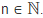That is, the set of ordered lists of n real numbers. One can also say that this is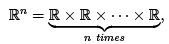the Cartesian product, deﬁned recursively. Given two elements

(x1 , · · · , xn ) and (y1, . . . , yn)

in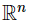, then the vector sum is simply the new vector

(x1 + y1, · · · , xn + yn).

Scalar multiplication is

a · (x1, · · · , xn) = (a · x1, · · · , a · xn).

It is a trivial matter to verify that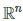, with these operations, is a vector space over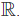• Let C0 ([0, 1],be the set of all continuous functions f : [0, 1] →This is a vector space with vector addition

(f + g)(x) = f (x) + g(x),

for all x ∈ [0, 1], deﬁning the new function (f + g) ∈ C0([0, 1],for all f , g ∈ C0([0, 1],Scalar multiplication is given by

(a · f )(x) = a · f (x)

for all x ∈ [0, 1].

Subspaces

Let V be a vector space over a ﬁeld F and let W ⊂ V be some subset. If W is itself a vector space over F , considered using the addition and scalar multiplication in V, then we say that W is a subspace of V. Analogously, a subset H of a group G, which is itself a group using the multiplication operation from G, is called a subgroup of G. Subﬁelds are similarly deﬁned.

Theorem 2. Let W ⊂ V be a subset of a vector space over the ﬁeld F .

Then W is a subspace of V ⇔ a · v + b · w ∈ W,

for al l v, w ∈ W and a, b ∈ F .

Proof. The direction ‘⇒’ is trivial.

For ⇐,’, begin by observing that 1. v + 1.w= v+w ∈ W, and a.v+0.w = a.v ∈ W, for all v, w ∈ W and a ∈ F. Thus W is closed under vector addition and scalar multiplication.

Is W a group with respect to vector addition? We have 0.v= O ∈ W, for v ∈ W; therefore the neutral element 0 is contained in W. For an  arbitrary v ∈ W we have

v + (−1) · v = 1 · v + (−1) · v
= (1 + (−1)) · v
= 0 · v
= 0.

Therefore (−1) · v is the inverse element to v under addition, and so we can simply write  (-1) .v = -v. the other axioms for a vector space can be easily checked.

The method of this proof also shows that we have similar conditions for subsets of groups or ﬁelds to be subgroups, or subﬁelds, respectively.

Offer running on EduRev: Apply code STAYHOME200 to get INR 200 off on our premium plan EduRev Infinity!

159 docs

,

,

,

,

,

,

,

,

,

,

,

,

,

,

,

,

,

,

,

,

,

,

,

,

;